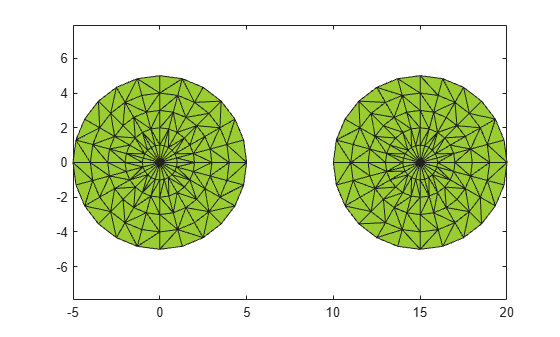# inShape

Determine if point is inside alpha shape

## Syntax

``tf = inShape(shp,qx,qy)``
``tf = inShape(shp,qx,qy,qz)``
``tf = inShape(shp,QP)``
``tf = inShape(___,RegionID)``
``````[tf,ID] = inShape(___)``````

## Description

example

````tf = inShape(shp,qx,qy)` returns logical `1` (`true`) values for the 2-D query points `(qx,qy)` that are within 2-D alpha shape `shp`. Otherwise, `inShape` returns values of logical `0` (`false`). The `qx` and `qy` arguments are numeric arrays whose corresponding elements specify the `(x,y)` query point coordinates.```
````tf = inShape(shp,qx,qy,qz)` tests whether the 3-D query points `(qx,qy,qz)` are within 3-D alpha shape `shp`.```
````tf = inShape(shp,QP)` specifies the 2-D or 3-D query point coordinates in a matrix with 2 or 3 columns.```
````tf = inShape(___,RegionID)` tests whether the query points are within a specific region of the alpha shape, using any of the previous syntaxes. `RegionID` is the ID for the region and `1` ≤ `RegionID` ≤ `numRegions(shp)`.```
``````[tf,ID] = inShape(___)``` also returns the IDs for the regions in the alpha shape that contain the query points. `ID` is `NaN` for query points that are not in the alpha shape.```

## Examples

collapse all

Create a set of 2-D points.

```th = (pi/12:pi/12:2*pi)'; x1 = [reshape(cos(th)*(1:5), numel(cos(th)*(1:5)),1); 0]; y1 = [reshape(sin(th)*(1:5), numel(sin(th)*(1:5)),1); 0]; x = [x1; x1+15;]; y = [y1; y1];```

Create and plot an alpha shape using an alpha radius of 2.5.

```shp = alphaShape(x,y,2.5); plot(shp)```Create a Cartesian grid of query points near the alpha shape.

`[qx, qy] = meshgrid(-10:2:25, -10:2:10);`

Check if the query points are inside of the alpha shape, and if so, plot them red. Plot the query points that lie outside of the alpha shape in blue.

```in = inShape(shp,qx,qy); plot(shp) hold on plot(qx(in),qy(in),'r.') plot(qx(~in),qy(~in),'b.')```## Input Arguments

collapse all

Alpha shape, specified as an `alphaShape` object. For more information, see `alphaShape`.

Example: `shp = alphaShape(x,y)` creates a 2-D `alphaShape` object from the `(x,y)` point coordinates.

Query point x-coordinates, specified as a numeric array.

Data Types: `double`

Query point y-coordinates, specified as a numeric array.

Data Types: `double`

Query point z-coordinates, specified as a numeric array.

Data Types: `double`

Query point coordinates, specified as a matrix with two columns (2-D) or a matrix with three columns (3-D).

• For 2-D, the columns of `QP` represent `x` and `y` coordinates, respectively.

• For 3-D, the columns of `QP` represent `x`, `y`, and `z` coordinates, respectively.

Data Types: `double`

ID number for region in alpha shape, specified as a positive integer scalar between `1` and `numRegions(shp)`.

An alpha shape can contain several smaller regions, depending on the point set and parameters. Each of these smaller regions is assigned a unique `RegionID`, which numbers the regions from the largest area or volume to the smallest. For example, consider a 3-D alpha shape with two regions. The region with the largest volume has a `RegionID` of 1, and the smaller region has a `RegionID` of 2.

Example: `shp.RegionThreshold = area(shp,numRegions(shp)-2);` suppresses the two smallest regions in 2-D alpha shape `shp`.

Data Types: `double`

## Output Arguments

collapse all

Status of the query points, returned as a logical array. The size of `tf` is equal to the size of the inputs that specify the query points (`qx`, `qy`, `qz`, or `QP`).

`inShape` returns logical `1` (`true`) values for points that are within the alpha shape or exactly on the boundary.

IDs of regions containing query points, returned as a numeric array. `ID` is the same size as `tf`.